StatLect

# Elementary column operations

All the theory of linear systems we have discussed so far (e.g., matrix form, equivalent systems, elementary row operations, row echelon form, Gaussian elimination) depends on the choice we have initially made of arranging the equations of the system vertically (one below the other) and writing their left- and right-hand sides as entries of two column vectors.

A parallel, and mostly identical, theory can be developed by arranging the equations horizontally (one besides the other) and writing their left- and right-hand sides as entries of two row vectors. In this parallel theory, which is briefly outlined below, elementary column operations (which are the counterpart of elementary row operations) play a key role. Furthermore, precisely defining column operations is worthwhile because several useful concepts in linear algebra rely on a unified treatment of row and column operations.## Systems in row form

Remember that a system oflinear equations inunknowns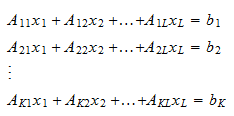can be represented in matrix form aswhereis thematrix of coefficients,is thevector of constants andis thevector of unknowns.

Thus, we have twocolumn vectors on the two sides of the equal sign:

•on the left-hand side;

•on the right-hand side.

By transposing both sides of the system, we can equivalently write it asso that the vector of unknownsis arow vector, the matrix of coefficientsis amatrix and the vector of constantsis arow vector.

In other words, we have tworow vectors on the two sides of the equal sign:

•on the left-hand side;

•on the right-hand side.

This is what we meant in the introduction by "arranging the equations horizontally, one besides the other".

Notice that:

• in the system, each row is an equation;

• in the system, each column is an equation.

Example Consider the systemThen,and we have twovectors on the two sides of the equal sign:Each row of the two vectors corresponds to an equation. By transposing everything, we getand we have twovectors on the two sides of the equal sign: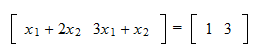Each column of the two vectors corresponds to an equation. To better visualize the correspondence between columns, we could write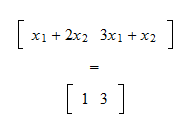## Column operations

When a system is written horizontally, we can obtain systems equivalent to it by performing elementary column operations:

1. multiplying a column by a non-zero constant;

2. adding a multiple of one column to another column;

3. interchanging columns.

These operations are completely analogous to the elementary row operations performed on systems written vertically.

1. directly on the rows of the system;

2. on the rows of the identity matrix; the system is then pre-multiplied by the resultant matrix.

The same is true of elementary column operations, who can be performed:

1. directly on the columns of the system;

2. on the columns of the identity matrix; the system is then post-multiplied by the resultant matrix.

This can be easily seen as follows. When the system is arranged vertically and the matrix obtained by performing elementary row operations is, then the equivalent system isIf we transpose both sides of the equation, we getwhereis the matrix obtained by performing column operations on the identity matrix (transposition transforms row operations into column operations).

Example Consider the system of two equations in three unknownsthat can be written in matrix form aswhere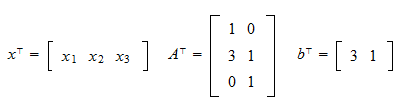Adding the first equation to the second, we obtain the equivalent systemthat can be written in matrix form aswhere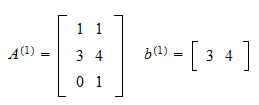We obtain the same result by 1) taking theidentity matrix2) adding its first column to the second:and 3) post-multiplyingandby: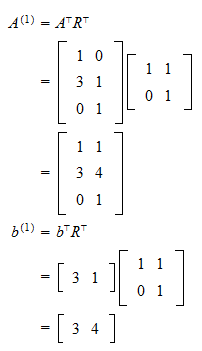## Column echelon form

Remember that systems arranged vertically are easy to solve when they are in row echelon form or reduced row echelon form. These forms have obvious counterparts for systems arranged horizontally: the systemis said to be in (reduced) column echelon form if and only if the systemis in (reduced) row echelon form.

Equivalently, the matrixis said to be in (reduced) column echelon form if and only if the matrixis in (reduced) row echelon form.

## Gaussian and Gauss Jordan elimination

Finally, Gaussian and Gauss Jordan elimination, the two algorithms used to transform a vertical system into an equivalent system in (reduced) row echelon form, can be used on a horizontal system with straightforward modifications: whenever an elementary row operation is necessary for the vertical system, we instead perform a column operation on the horizontal system.

## Solved exercises

Below you can find some exercises with explained solutions.

### Exercise 1

Write the systemhorizontally.

Solution

The system is### Exercise 2

Write the matrixthat allows us to sum twice the first column of the system in the previous exercise to the second column.

Solution

The matrixis obtained by performing the elementary column operation on theidentity matrix: